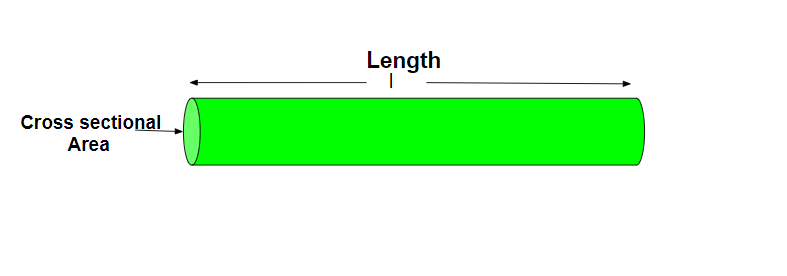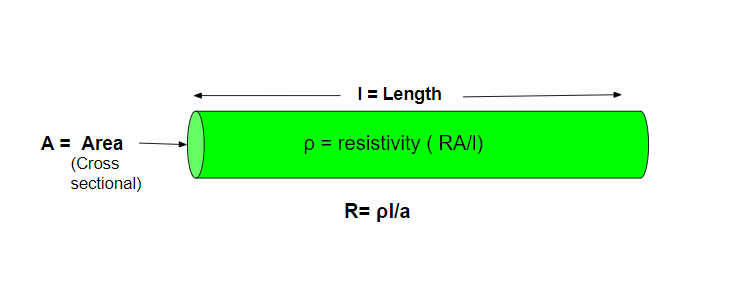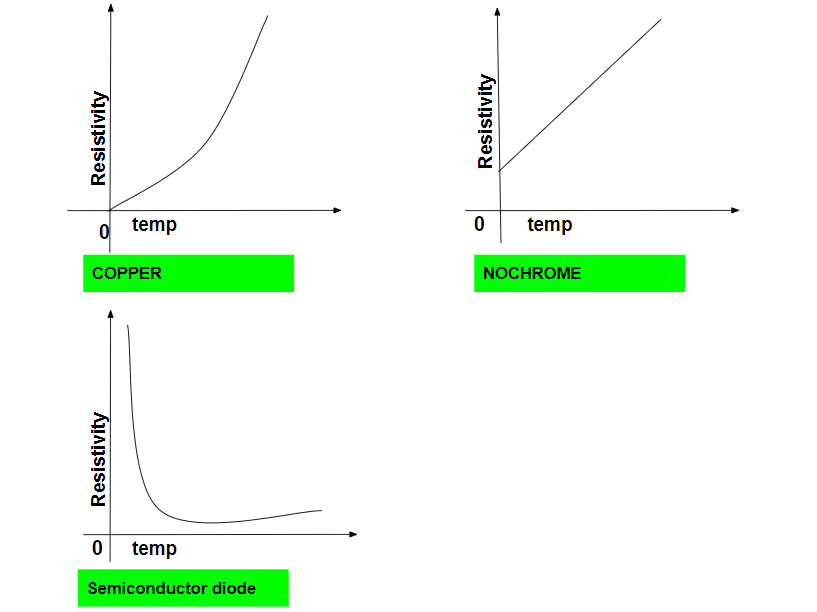Open In App

# Resistivity Formula

When a potential difference is applied across an object (conductor), the electrons start moving, which produces a current in the object. During this movement of electrons, they collide with other electrons too, which causes some opposition to the flow of electrons, this phenomenon of opposing the flow of electrons is known as Resistance(R). Its SI unit is the ohm(Ω)

Factors affecting the resistance• Resistance  R of a conductor is directly proportional to the length of the conductor

R ∝ l ⇢ (1)

• The resistance of a conductor is inversely proportional to the area of cross-section when the conductor is in a uniform shape.

R ∝ 1/A ⇢ (2)

• The resistance of a conductor also depends upon the nature of the conductor as well as the temperature of the conductor. So, by combining equations (1) and (2),

R ∝ l/A

R = ρl/A

ρ(rho) is constant which is called resistivity.

### Resistivity Formula

The resistivity of a material is defined as the resistance of a conductor of material per unit length and per unit cross-sectional area at a particular temperature

OR

It is the property of a material that demonstrates how strongly the material resists or conducts electric current. It is denoted by ρ which is called rho. The formula for resistivity is given as,

•  ρ = (RA)/l ⇢ (where ‘R’ is resistance, ‘A’ is the Area of cross section ,’l’ is the length)
•  ρ = 1/σ ⇢ (σ is the conductivity)Derivation of resistivity

Given: R = ρl/a

Where, R – Resistance,

ρ – Resistivity

l – length of material

A – Area of cross-section

Rearranging the above equation,

RA = ρl

ρl = RA

ρ = (RA)/l

SI unit of resistivity

SI unit of R = ohm

SI unit of  A = m2

SI unit of  l = m

SI unit of ρ = (ohm × m2)/m

ρ = ohm m

SI unit of resistivity is ‘ohm meter(Ωm)’

Factors affecting the resistivity

• The resistivity of a conductor is directly proportional to its resistance [ρ ∝ R].
• The resistivity of a conductor is directly proportional to its uniform cross-sectional area. [ρ ∝ A]
• The resistivity of a conductor is inversely proportional to its length. [ρ ∝ 1/l]
• Resistivity also depends upon the nature of the material and temperature as well.

### Sample problems

Question 1: Calculate the resistivity of a wire whose length is 30cm and the area is 0.9 m2. The resistance of that wire is 5Ω?

Solution:

R = 5Ω

l = 30 cm = o.3 m

A = 0.9m2

ρ = RA / l

ρ = (5 × 0.9)/0.3  Ωm

ρ = 15  Ωm

Therefore resistivity will be 15 Ωm

Question 2: Given that conducting of material is 4 ohm-1m-1. Calculate resistivity?

Solution:

Conductivity (σ ) = 4 ohm-1m-1

ρ   = 1/ σ

ρ  = 1/4 ohm m

ρ  = 0.25 ohm m

So, resistivity will be 0.25 ohm m

Question 3: Why alloys are used for making standard resistors?

Solution:

Alloys have a high value of resistivity  as well its has low temperature coefficient of resistance.

Question 4: Name the element which has the highest resistivity and the metal which has the lowest resistivity?

Solution:

Nichrome has the highest resistivity (1.50 × 10-6 Ωm)

Question 5: Identify which has the best conductivity as given that the resistivity of copper, constantan, and silver are 1.74 × 10-8Ωm, 39.1 × 10-8Ωm, and 1.6 × 10-8Ωm respectively?

Solution:

Conductivity = 1/ resistivity

Silver has the best conductivity as it has the lowest resistivity

Question 6: Calculate the resistance of a metal wire of length 3m and 0.6 of diameter having resistance is 60 Ω?

Solution :

R = 60

Diameter = 0.6 m

Area of cross section = πr2

A = 3.14 × (0.3 × 0.3)m2

A = 0.2826

Length (l) = 3m

ρ = RA / l

ρ = (60 × 0.2826)/3

ρ = 5.652 Ωm

Question 7: Plot the graph for resistivity versus temperature for copper, nichrome, semiconductor diode.